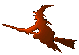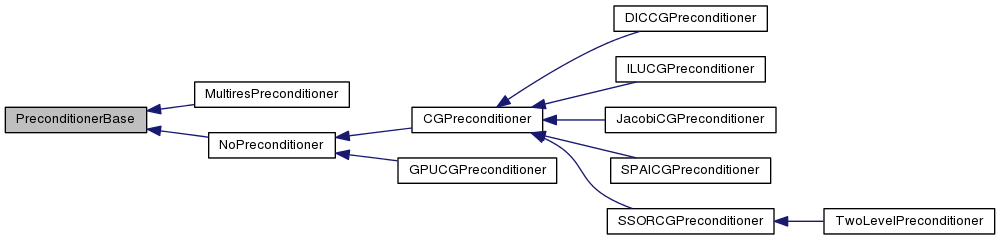Hex  1.0 Hydrogen-electron collision solver
PreconditionerBase Class Referenceabstract

Preconditioner template. More...

#include <preconditioners.h>

Inheritance diagram for PreconditionerBase:[legend]

## Public Member Functions

virtual void setup ()=0
Initialize the preconditioner. More...

virtual void update (double E)=0
Update the preconditioner for the next energy. More...

virtual void rhs (cArrayView chi, int ienergy, int instate) const =0
Return the right-hand side. More...

virtual void multiply (const cArrayView p, cArrayView q) const =0
Multiply by the matrix equation. More...

virtual void precondition (const cArrayView r, cArrayView z) const =0
Precondition the equation. More...

## Detailed Description

This interface class declares all necessary methods that a valid preconditioner object needs to implement. The preconditioner thus needs to be able to multiply a vector by the matrix of the set of equations that is to be solved and, of course, to precondition the solution by solving the auxiliary preconditioner equation $$\mathbf{M}\mathbf{z} = \mathbf{r}$$.

## Member Function Documentation

 virtual void PreconditionerBase::multiply ( const cArrayView p, cArrayView q ) const
pure virtual

This function implements matrix multiplication by the matrix of the set of equations that is to be solved.

 virtual void PreconditionerBase::precondition ( const cArrayView r, cArrayView z ) const
pure virtual

This function preconditions the equation, solving the preconditioner equation

$\mathbf{M}\mathbf{z} = \mathbf{r} \ .$

It may use the MPI environment.

pure virtual
 virtual void PreconditionerBase::rhs ( cArrayView chi, int ienergy, int instate ) const
pure virtual
 virtual void PreconditionerBase::setup ( )
pure virtual

This function contains all computation intensive preparations for the preconditioner, e.g. computation of radial integrals. It may use only SMP environment.

 virtual void PreconditionerBase::update ( double E )
pure virtual

This function updates the preconditioner for another right hand side. It may use the MPI environment.

The documentation for this class was generated from the following file: